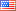##### Shrink: An Efficient Construction Algorithm for Minimum Vertex Cover Problem
نوع المنشور
بحث أصيل
المؤلفون

The minimum vertex cover (VC) problem is to find a minimum number of vertices in an undirected graph such that every edge in the graph is incident to at least one of these vertices. This problem is a classical NP-hard combinatorial optimization problem with applications in a wide range of areas. Hence, there is a need to develop approximate algorithms to find a small VC in a reasonable time. This paper presents a new construction algorithm for the minimum VC problem. Extensive experiments on benchmark graphs show that the proposed algorithm is extremely competitive and complementary to existing construction algorithms for minimum VC problem.

المجلة المحكمة
العنوان
Information Sciences Letters
الناشر
Natural Sciences Publishing
بلد الناشر
الولايات المتحدة الأمريكيةIndexing
Scopus
معامل التأثير
None
نوع المنشور
Both (Printed and Online)
المجلد
10
السنة
2021
الصفحات
255-261